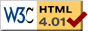### Journée-séminaire de combinatoire

#### (équipe CALIN du LIPN, université Paris-Nord, Villetaneuse)

Le 14 novembre 2013 à 11h15 en B107, Vincent Vargas nous parlera de : Critical and dual Gaussian multiplicative chaos

Résumé : The theory of Gaussian multiplicative chaos enables to make sense of random measures defined formally by the exponential of a Gaussian Free Field (and more generally any logarithmically correlated field in all dimensions). These random measures are conjectured to be the scaling limit of random planar maps coupled to a CFT and conformally mapped to the sphere or the upper half plane. The construction of the measures involve a parameter gamma related to the central charge c of the CFT. The classical construction does not settle the question of constructing the measure for c=1 or equivalently gamma=2 as it yields a vanishing measure. In this talk, I will explain the appropriate construction and some properties of Gaussian multiplicative chaos at criticality, i.e. for gamma=2. If time permits, I will also explain the construction of the dual measure (gamma larger than 2) and how to make sense of the KPZ equation in this context.

 Dernière modification : Monday 24 January 2022Contact pour cette page : Cyril.Banderier at lipn.univ-paris13.fr